# Comparative Study Between Two Control Techniques Applied on the Permanent Magnet Synchronous Machine (PMSM)

Comparative Study Between Two Control Techniques Applied on the Permanent Magnet Synchronous Machine (PMSM)

Department of Electrical Engineering, Faculty of Technology, University of M’sila, Algeria, 28000 M’sila

LEB – Research Laboratory, Department of Electrical Engineering, Batna02 University Algeria, 05000 Batna

Corresponding Author Email:
Page:
87-94
|
DOI:
https://doi.org/10.18280/ama_c.742-407
3 April 2019
|
Accepted:
25 August 2019
|
Published:
30 December 2019
| Citation

OPEN ACCESS

Abstract:

In this article, we present a comparative study between two control techniques applied on the Permanent Magnet Synchronous Machine (PMSM), namely vector control and fuzzy logic. This comparison is based on three criteria: qualitative, quantitative and robust during the transient and permanent operation of the system. The latter comprises a machine is driven through the stator variables by two bidirectional converters. In the first part, we have presented the individual modeling of the global chain (PMSM, Inverter, and Rectifier). Then we presented and developed the two commands techniques to control the speed and the torque produced by this machine. The results of this study made it possible to evaluate the performance of these controls.

Keywords:

bidirectional converter, comparative study, fuzzy logic control, modelling, permanent magnet synchronous machine, vector control

1. Introduction

Permanent magnet synchronous machines have been booming in recent years. It is thanks to the improvement of the permanent magnet’s qualities more precisely with the help of the rare earths [1, 2]. The strengths of this type of machine are many, among which we can mention: robustness, low inertia, high mass torque, high efficiency, maximum top speed and low maintenance cost [3, 4]. Moreover, permanent magnets have undeniable advantages: on the one hand, the inductive flux is created without loss of excitation and on the other hand, the use of these materials will allow to deviate significantly from the usual sizing constraints machines and therefore to increase the power mass significantly [5, 6]. This type of machine enjoys a remarkable reputation in several sectors: servomotor, land transport (rail), embedded systems, and wind energy [7, 8]. Despite all the advantages of the PMSM, its control remains one of the most complex, because its mathematical model is nonlinear and strongly coupled.

Among the efficiency factors of the PMSM is the evolution of microelectronics and micro informatics that have enabled the development of electronic devices based on high frequency and high-power components controlled by programmable microprocessors. These in turn enable the implementation of various more efficient control techniques that will be adapted to the system around a specific operating point in order to establish appropriate optimization algorithms with a minimum of disturbances on the distribution grid [9-11].

The main objective of our work is to apply two control techniques to the permanent magnet synchronous machine namely: the vector control and the control by the logic flue, afterwards we have arrived a comparative study between these commands in order to choose the best suited from a qualitative, quantitative and robust point of view.

The rest of the paper is structured as follows. The next section gives the different system component models (PMSM, Inverter, and Rectifier). In Section 2, proposed an efficient PMSM speed controller based on vector algorithm to ensure a high-performance control for speed. In Section 3, a fuzzy logic control applied to the whole system model is given. In Section 4, the comparative study between the different laws of commands proposed in this work. Finally, conclusion of the present work is drawn.

2. Modelling of the Individual Components of System

In this context will be devoted to the individual modeling of our system. We will start with the modeling of the permanent magnet synchronous machine in the Park reference linked to the rotating field and its stator power supply (two-level inverter) which will allow the application of commands intended to control the speed and the torque generated by the rotor of the machine. Then the inverter power modeling which is a three-phase converter (two-level rectifier) and its control to improve the power factor on the grid side and ensure the setting of the Direct Current (DC) bus.

2.1 Modelling of the permanent magnet synchronous machine

The permanent magnet synchronous machine is a very complex nonlinear system. To have a good control on this machine in the different operating modes, one needs a precise and demanding mathematical modeling to represent its behavior in a satisfactory and real way.

$\left\{ \begin{array}{*{35}{l}} \frac{d}{dt}{{I}_{d}}=\frac{1}{{{L}_{d}}}\left( {{V}_{d}}-{{R}_{s}}{{I}_{d}}+\omega {{L}_{q}}{{I}_{q}} \right) \\ \frac{d}{dt}{{I}_{q}}=\frac{1}{{{L}_{qs}}}\left( {{V}_{q}}-{{R}_{s}}{{I}_{q}}-\omega {{L}_{d}}{{I}_{d}}-\omega {{\varnothing }_{f}} \right) \\ {{C}_{e}}=\frac{3}{2}p\left[ \left( {{L}_{d}}-{{L}_{q}} \right){{I}_{d}}{{I}_{q}}+{{\varnothing }_{f}}{{I}_{q}} \right] \\ {{C}_{e}}-{{C}_{r}}-f\Omega =\text{j}\frac{d}{dt}\Omega \\\end{array} \right.$   (1)

In the state space representation, the system is given as:A classical modelling of the Permanent Magnet Synchronous Machine in the Park reference frame is used. The voltage and flux equations of the PMSM [12-14]:

$[\dot{X}]=[A][X]+[B][V]$    (2)

[X]: State vector; [A]: Fundamental matrix that characterizes the system; [B]: Input Matrix; [V]: Command vector.

$\frac{d}{d t}[X]=[A][X]+[B][V]$    (3)

With: [X]=[Id Iq]T; [V]=[Vd Vq $\varnothing_f$]T

$\left[ \begin{matrix} {{I}_{d}} \\ {{I}_{q}} \\\end{matrix} \right]=\left[ \begin{matrix} \frac{-{{R}_{s}}}{{{L}_{d}}} & \omega \frac{{{L}_{q}}}{{{L}_{d}}} \\ -\omega \frac{{{L}_{d}}}{{{L}_{q}}} & \frac{-{{R}_{s}}}{{{L}_{q}}} \\\end{matrix} \right]\left[ \begin{matrix} {{I}_{d}} \\ {{I}_{q}} \\\end{matrix} \right]+\left[ \begin{matrix} \frac{1}{{{L}_{d}}} & 0 & 0 \\ 0 & \frac{1}{{{L}_{q}}} & -\frac{\omega }{{{L}_{q}}} \\\end{matrix} \right]\left[ \begin{matrix} {{V}_{d}} \\ {{V}_{q}} \\ {{\varnothing }_{f}} \\\end{matrix} \right]$   (4)

$[A]=\left[ \begin{matrix} \frac{-{{R}_{s}}}{{{L}_{d}}} & 0 \\ 0 & \frac{-{{R}_{s}}}{{{L}_{q}}} \\\end{matrix} \right]+\left[ \begin{matrix} 0 & \frac{{{L}_{q}}}{{{L}_{d}}} \\ -\frac{{{L}_{d}}}{{{L}_{q}}} & 0 \\\end{matrix} \right]\omega =\left[ {{A}_{1}} \right]+\omega \left[ {{A}_{2}} \right]$   (5)

$[B]=\left[ \begin{matrix} \frac{1}{{{L}_{d}}} & 0 & 0 \\ 0 & \frac{1}{{{L}_{q}}} & 0 \\\end{matrix} \right]+\left[ \begin{matrix} 0 & 0 & 0 \\ 0 & 0 & -\frac{1}{{{L}_{q}}} \\\end{matrix} \right]\omega =\left[ {{B}_{1}} \right]+{{\omega }_{\cdot }}\left[ {{B}_{2}} \right]$    (6)

2.2 Modelling of the stator side converter (Inverter)

## 7.1.png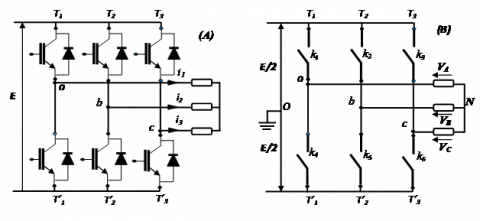Figure 1. Schematic diagram of the stator side converter

Converters associated with alternative current (AC) machines (inverters) are nowadays very widely used in industrial drive systems. The stator of the PMSM is powered by a two levels voltage inverter. This inverter is equipped with several semiconductor devices controlled at the opening and closing which can be either MOSFET transistors or IGBTs associated with diodes head to tail. The main objectives of this converter are: to wave the voltage of the DC bus to supply it to the stator winding and to allow the application of the commands to control the mechanical power generated (Speed; Torque) by the rotor of this machine [15-17].

The converter used is a simple three-phase inverter on two levels.

The equations for simple voltages at three phases are:

$\left\{\begin{array}{l}V_{A}=V_{A O}+V_{O N} \\ V_{B}=V_{B O}+V_{O N} \\ V_{C}=V_{C O}+V_{O N}\end{array}\right.$    (7)

Knowing that the system of three-phase statoric voltages is symmetrical.

$V_{A O}+V_{B O}+V_{C O}+3 * V_{O V}=0$    (8)

$V_{O N}=-\frac{1}{3}\left(V_{A O}+V_{B O}+V_{C O}\right)$    (9)

By replacing (9) in (7), we obtain the following system:

$\left\{\begin{array}{l}V_{A O}=\frac{2}{3} V_{A O}-\frac{1}{3} V_{B O}-\frac{1}{3} V_{C O} \\ V_{B O}=-\frac{1}{3} V_{A O}+\frac{2}{3} V_{B O}-\frac{1}{3} V_{C O} \\ V_{C O}=-\frac{1}{3} V_{A O}-\frac{1}{3} V_{B O}+\frac{2}{3} V_{C O}\end{array}\right.$    (10)

$\left[\begin{array}{c}V_{A} \\ V_{B} \\ V_{C}\end{array}\right]=\frac{1}{3}\left[\begin{array}{ccc}2 & -1 & -1 \\ -1 & 2 & -1 \\ -1 & -1 & 2\end{array}\right]\left[\begin{array}{c}V_{A O} \\ V_{B O} \\ V_{C O}\end{array}\right]$     (11)

Thanks to the successive opening and closing of the switches, the inverter generates an alternating voltage formed of a succession of rectangular slots.

$\left\{\begin{array}{l}V_{A O}=\frac{E}{2} S_{1} \\ V_{B O}=\frac{E}{2} S_{2} \\ V_{C O}=\frac{E}{2} S_{3}\end{array}\right.$    (12)

\left\{ \begin{align} & {{S}_{1}}=1\text{ }Si\text{ }{{k}_{1}}\text{ ferme si nom }{{S}_{1}}=-1\text{ } \\ & {{S}_{2}}=1\text{ }Si\text{ }{{k}_{2}}\text{ ferme si nom }{{S}_{2}}=-1 \\ & {{S}_{3}}=1\text{ }Si\text{ }k3\text{ ferme si nom }{{S}_{3}}=-1 \\ \end{align} \right.   (13)

By replacing (12) in (11), we will have the following system:

$\left[\begin{array}{c}V_{A} \\ V_{B} \\ V_{C}\end{array}\right]=\frac{E}{6} \cdot\left[\begin{array}{ccc}2 & -1 & -1 \\ -1 & 2 & -1 \\ -1 & -1 & 2\end{array}\right]\left[\begin{array}{c}S_{1} \\ S_{2} \\ S_{3}\end{array}\right]$     (14)

2.3 Modelling and control of the grid side converter (Rectifier)

Our system uses the PWM (Pulse-width modulation) rectifier for connection to the power grid. The rectifier has the same design as that of the inverter previously established. The advantage of the converter on the grid side, in addition to the bidirectionality of power, makes it possible to control the constant DC bus voltage, and to set the reference reactive power to a zero value so as not to impair the quality of the grid (power factor of the unitary grid).

Figure 2 shows the structure of a three-phase PWM rectifier, which can be broken down into three parts: the source, the converter and the load [15-17].

## 7.2.pngFigure 2. Structure of the PWM rectifier

2.3.1 Modelling of the grid side converter

The AC source model and the rectifier model are given by the state space representation:

$\frac{d}{d t}\left[\begin{array}{c}i_{1} \\ i_{2} \\ i_{3}\end{array}\right]=\left[\begin{array}{ccc}-\frac{R}{L} & 0 & 0 \\ 0 & -\frac{R}{L} & 0 \\ 0 & 0 & -\frac{R}{L}\end{array}\right]\left[\begin{array}{c}i_{1} \\ i_{2} \\ i_{3}\end{array}\right]+\frac{1}{L}\left[\begin{array}{c}V_{1}-V_{a n} \\ V_{2}-V_{b n} \\ V_{3}-V_{c n}\end{array}\right]$    (15)

The converter:

$\left[\begin{array}{c}V_{A} \\ V_{B} \\ V_{C}\end{array}\right]=\frac{U_{c}}{3} \cdot\left[\begin{array}{ccc}2 & -1 & -1 \\ -1 & 2 & -1 \\ -1 & -1 & 2\end{array}\right]\left[\begin{array}{c}S_{1} \\ S_{2} \\ S_{3}\end{array}\right]$    (16)

In addition, the rectified current is given by:

$i_{s}=\left[\begin{array}{lll}S_{1} & S_{2} & S_{3}\end{array}\right]\left[\begin{array}{l}i_{1} \\ i_{2} \\ i_{3}\end{array}\right]$    (17)

The output voltage is governed by:

$\frac{d U_{C}}{d t}=\frac{1}{C}\left(i_{s}-i_{L}\right)$     (18)

## 7.3.png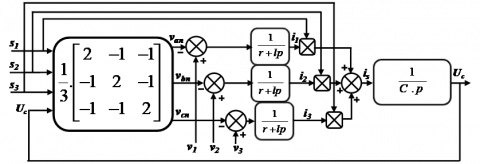Figure 3. Schematic diagram of grid side converter

2.3.2 Grid side converter controller design

The objective of the grid side converter is to regulate the DC link voltage and to set a unit power factor. The equations allowing the expression of the input AC voltage Vp(d,q) to the rectifier in the (d,q) frame as a function of the voltages at the common connection point (CCP), V(d,q)are given by the following equations:

$\left\{\begin{array}{l}V_{p d}=V_{d}-R \cdot i_{d}-L \frac{d i_{d}}{d t}+L w \cdot I_{q} \\ V_{p q}=V_{q}-R \cdot i_{q}-L \frac{d i_{q}}{d t}-L w \cdot I_{d}\end{array}\right.$    (19)

The real and reactive powers are expressed by:

$\left\{\begin{array}{l}P=\frac{3}{2}\left[V_{d} I_{d}+V_{q} I_{q}\right] \\ Q=\frac{3}{2}\left[V_{q} I_{d}+V_{d} I_{q}\right]\end{array}\right.$    (20)

This system can be written in the following matrix form:

$\left[ \begin{array}{*{35}{l}} P \\ Q \\\end{array} \right]=\frac{3}{2}\cdot \left[ \begin{matrix} {{V}_{d}} & {{V}_{q}} \\ {{V}_{q}} & {{V}_{d}} \\\end{matrix} \right]\left[ \begin{array}{*{35}{l}} {{I}_{d}} \\ {{I}_{q}} \\\end{array} \right]$    (21)

Equation (22) allows us processing the reference currents $\left(I_{d}^{*}, I_{q}^{*}\right)$ knowing the active and reactive powers references $\left(P^{*}, Q^{*}\right)$.

$\left[\begin{array}{l}I_{d}^{*} \\ I_{q}^{*}\end{array}\right]=\frac{2}{3\left(V_{d}^{2}+V_{q}^{2}\right)}\left[\begin{array}{cc}V_{d} & V_{q} \\ V_{q} & -V_{d}\end{array}\right]\left[\begin{array}{l}P^{*} \\ Q^{*}\end{array}\right]$    (22)

where, Vd, Vq, are the actual measured grid voltages.

(1) The desired reference voltage of the DC bus Ucref is compared to that measured across the capacitor Ucmes.

(2) The Integral Proportional Corrector (PI) makes it possible to maintain the DC bus voltage at a desired constant value, and generates the reference current Icref.

(3) The active power needed to charge this capacitor Pref is obtained by multiplying the rectified current Ired_ref  by the measured voltage Ucmes.

(4) The reference reactive power Qref will be maintained at zero.

(5) The reference currents are obtained from the measured voltages and reference powers.

(6) The reference currents will be compared with the measured currents.

(7) The current comparison errors are set by PI controllers which generate reference voltages which will in turn be compared with the measured voltages.

(8) Voltage comparison errors generate voltages that will be compared in turn with the voltages of the filter.

(9) Current comparison errors generate control voltages used to switch the six rectifier switches to close and open. The block diagram of the regulation is then represented by the Figure 4 below.

## 7.4.png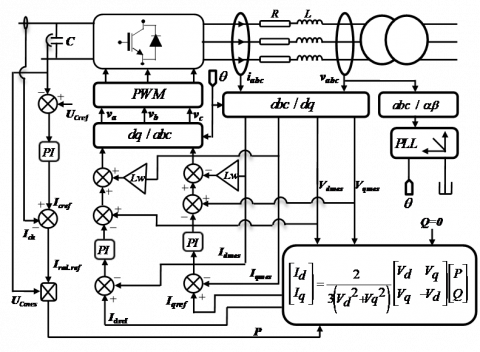Figure 4. Block diagram of the grid side converter control

3. Control of the Speed Produced by PMSM

To obtain an optimal quality of speed produced by PMSM, it is necessary to apply adequate optimization control techniques allowing the control of the speed generated by the rotor of this machine. From here, we propose two command techniques:

(1) Vector control based on PI controllers;

(2) Fuzzy logic control based on flue regulators.

3.1 Vector control of the speed delivered by PMSM

This command is one of the methods used to optimize the quality of control of the mechanical power produced by the PMSM. In this technique, the stator current vector will be decomposed into two components, one providing flow control and the other acting on the torque and making its dynamics identical to that of the separately excited DC machine [18-19].

The model of the PMSM is given by the following equations:

$\left\{\begin{array}{l}V d s=\left(R_{s}+L_{d} P\right) I_{d}-\omega L_{q} I_{q} \\ V q s=\left(R_{s}+L_{q} P\right) I_{q}+\omega L_{d} I_{d}+\omega \varnothing_{f} \\ \mathrm{j} \frac{d}{d t} \omega=C e-C r-f \omega\end{array}\right.$   (23)

With: $\omega=p \cdot \omega_{r}$

$C e=\frac{3}{2} p\left[\left(L_{d}-L_{q}\right) I_{d} \cdot I_{q}+\varnothing_{f} \cdot I_{q}\right]$    (24)

The principle of the vector control of the PMSM by orientation of the rotor flow is intended to orient the rotor flow along the axis d. This strategy consists in keeping the axis d constantly aligned with the flux vector of the magnet. The reference for the current is id kept at zero. The reference for the current Iq is determined by means of an Integral-Proportional Corrector (IP) of speed. This regulator has the advantage of not introducing a zero in the closed-loop transfer function while guaranteeing a zero static error. In this command, we used non-linear decoupling to close the Park current regulation loop through Proportional-Integral (PI) controllers [20-21] and .

${{I}_{d}}=0\to {{I}_{q}}={{I}_{s}}$    (25)

The equation system of PMSM becomes:

$\left\{\begin{array}{l}V d s=-\omega L_{q} I_{q} \\ V q s=\left(R_{s}+L_{q} P\right) I_{q}+\omega \varnothing_{f} \\ C e=\frac{3}{2} p \varnothing_{f} I_{q}\end{array}\right.$   (26)

As the flow $\emptyset_{f}$ fest constant, the torque is directly proportional to Iq.

so,

$Ce=K{{t}_{q}}$    (27)

where,

$Kt=\frac{3}{2}p\varnothing f$    (28)

3.2 Fuzzy logic control of the speed produced by PMSM

Fuzzy logic control is a powerful nonlinear control that has been analyzed by many researchers, especially in recent years. Fuzzy logic control has enhanced robustness and has been successfully applied to the control of the permanent magnet synchronous machine (PMSM) [23-24]. We will apply this non-linear control to control the quality of mechanical power (speed and torque) produced by the PMSM. To do this, we will use the structure of the vector control while replacing the PI regulator by a fuzzy regulator (regulator type Mamdani seven classes).

For the speed loop, the input variables are:

The speed error

$e_{I}=\Omega^{r e f}-\Omega$     (29)

The variation of the speed error

$\Delta e_{I}=e_{I}(k)-e_{I}(k-1)$    (30)

The majority of fuzzy controllers for single-chip systems consist of fuzzy controller inputs that are usually the error (the difference between the setpoint and the output of the process) and its variation (system dynamics translation). The majority of developed controllers use the simple scheme proposed by Mamdani, as shown in the following Figure 5 [25, 26]:

## 7.5.png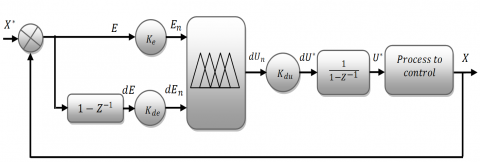Figure 5. Block diagram of the PI-fuzzy type controller structure [26, 27]

According to the diagram above, the fuzzy control system (fuzzy controller) consists essentially of:

(1) A calculation block of the variation of the error over time.

(2) Scale factors $\left(k_{e} k_{d e}, e t k_{d u}\right)$ that are normalization and denormalization gains. The appropriate choice of the latter makes it possible to guarantee the stability and the improvement of the targeted dynamic and static performances of the system to be regulated; moreover, the gains of entries $\left(k_{e}, k_{d e}\right)$  reduce the physical quantities of entries in a range of variation called universe of speeches.

(3) From a block of fuzzification of the error and its variation. For the choice of the form of the membership functions, we have opted for the triangular and trapezoidal shapes as shown in the Figure 6. The choice of linguistic variables is represented by: Negative Big (NB), Negative Medium (NM), Negative Small (NS), Zero (ZR), Positive Small (PS), Positive Medium (PM), and Positive Big (PB).

(4) A block of defuzzification of the variation of the command. The inference mechanism outputs that are fuzzy variables must be converted back to real output quantities so that the system can use them. In this step, a real value of the output variable is obtained by using the center of gravity method [28-29].

(5) The inference rules for determining the output variable for the speed setting grouped in the table below. The inference method used is the "min-max" method of Mamdani:

-Operator AND: formation of the minimum.

-Operator OR: formation of the maximum.

-Implication THEN: formation of the minimum.

-Aggregation: formation of the maximum.

(6) An integrator block used to integrate the variation of the resulting numerical control and its application into the system we want to control.

Table 1. Decision rules table for the speed controller

 The Control Error Nb NM Ns Zr Ps PM Pb Rate of change of error Nb NB NB NB NB ZR ZR ZR NM NB NB NM NM ZR ZR ZR Ns NB NB NS NS PS PS PM Zr NB NM NS ZR PS PM PB Ps NM NS NS PS PS PB PB PM ZR ZR ZR PM PM PB PB Pb ZR ZR ZR PB PB PB PB

## 7.6.png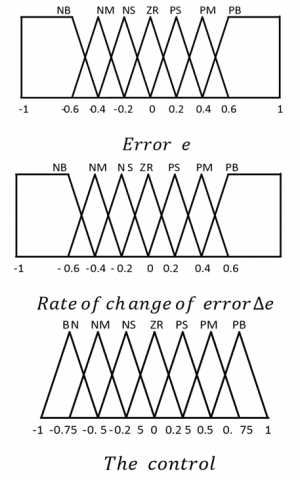Figure 6. The membership functions used by the control

4. Comparative Study of the Control Proposed

To examine the two control laws developed and synthesized on our system considered in this document, we will present a comparative study between these techniques. This study was repeated under the same conditions.

The goal in this part is to make a comparison between the two commands that we presented in our work. This comparison is made from a series of tests that we performed during the transient and permanent operation of the system:

The first test we have done is based on the influence of the set speed variation and the external variation (resisting torque), this comparison is called: qualitative comparison

The second test we have defined includes two criteria; one according to the applied command, which one can consider as an energetic criterion, the other according to the static error of the speed. This comparison is called quantitative comparison.

The last test we performed is to vary the parameters of the machine used, because, in reality, they are subject to variations caused by different physical phenomena such as (saturation of inductances, heating of resistors, etc....). This comparison is called: robustness comparison.

4.1 Qualitative comparison

4.1.1 Speed echlon variation test

This comparison is based on the observation of the results of simulations obtained by the application of the two control techniques developed on our machine. The setpoint of the proposed speed is given as a rung while the machine is rotated at a two fixed speeds. This test is carried out under the same conditions, namely:

(1) Machine runs at a fixed speed 314 rad/s between instants: t=0s and t=1s.

(2) Machine runs at a fixed speed -314 rad/s between instants: t=1s and t=2s.

(3) The resisting torque always keep zero for this test.

(4) The sampling period and the simulation time are fixed.

## 7.7.png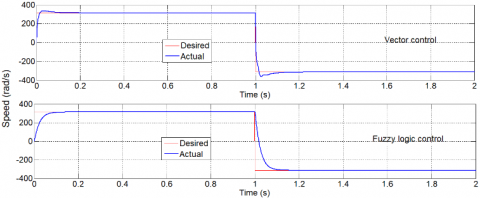Figure 7. Mechanical speed for reversal of direction of rotation at t=1s for vector control and fuzzy logic control

From the simulation results shown in the previous Figure 7 and 8, it is clear that the two commands have performances namely: the measured speed follows their new reference with tracking errors are low with acceptable exceedances, the times of the responses that characterize the transitional regime and the change of the instructions are weak. Also, a remarkable improvement of the results obtained by the fuzzy logic compared to the vector control is observed, namely:

(1) A zero overshoot in the transient regime for the control by the fuzzy logic against the vector control an overshoot is observed.

(2) A slower response time for the transient regime (0.1 for fuzzy logic control versus 0.05 for vector control); the same for the time of change of instructions.

(3) An exponential convergence of the errors towards zero between the values of setpoints and those measured.

(4) A remarkable minimization of oscillations of the regulated quantities and a fast regain.

## 7.8.pngFigure 8. Electromagnetic torque for reversal of direction of rotation at t=1s for vector control and fuzzy logic control

4.1.2 External variation test

(1) Machine runs at a fixed speed 314 rad/s between instants: t=0s and t=2s.

(2) Application of a resistant torque of 6 N.m between instants t=0.5s and t=1.5s.

(3) The sampling period and the simulation time are fixed.

## 7.9.png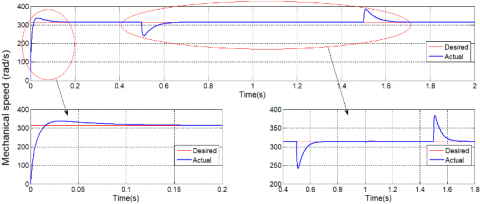Figure 9. Mechanical speed with application of a resisting torque of 6 (N.m) between times t=0.5s and t=1.5s for vector control

## 7.10.png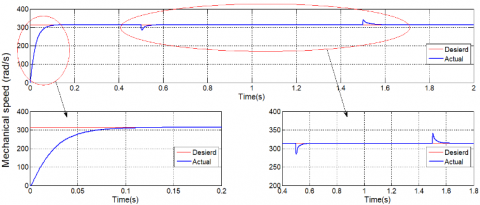Figure 10. Mechanical speed with application of a resistive torque of 6 (N.m) between instants t=0.5s and t=1.5s for by fuzzy logic control

The responses obtained with the two types of control show that the fuzzy logic controlled system is more efficient with respect to the variation of the resistive torque with respect to the vector control. Where we notice that:

When applying the load (Cr=6 Nm at t=0.5 s) a decrease in speed is observed which is rejected for both commands, by comparison the decrease in speed in the fuzzy control is minimal and faster than by report that of the vector control.

When removing the load (Cr=0 Nm at t=0.5 s) an increase in speed is observed which is rejected for both commands, by comparison the decrease in speed in the fuzzy control is minimal and faster than compared to that of the vector control.

4.2 Quantitative comparison

The second test based on two criteria: energetic J1 and static J2. The first is a function of the command applied, while the second is a function of the resulting error. The results were obtained under the same conditions. The energy J2 and precision criteria J2 are defined by:

${{J}_{1}}=\frac{1}{2}\sum\limits_{k=1}^{P}{\left( \begin{array}{*{35}{l}} u & u \\\end{array} \right)}$    (31)

${{J}_{2}}=\frac{1}{2}\sum\limits_{k=1}^{P}{\left( \begin{array}{*{35}{l}} e & e \\\end{array} \right)}$   (32)

J1: The value of the static error between the reference variable and the measured one.

J2: The power value necessary to apply control.

The objective in this part is the comparison of the two controls laws quantitatively (in figures); to highlight the performance of each of them. The values of the static error J1 and that of the required command J2 are calculated in the time interval [0s 5s] for two commands.

Simulation results presented in the above table show clearly that the vector control is the most performing from the minimization point of the energy criterion which gives us the lowest values of J1 (J1=2.7474e+04) for the mechanical speed, then control by fuzzy logic. However, as regards the second precision criterion, it is notice that it is the dark logic command which gives the lowest value of J2 (J2=2.7851e+04) for the mechanical speed, then the vector control.

Table 2. Comparative study of the commands developed for the considered system

 G-C Criterion Commands developed for the considered system PI regulator PI fuzzy regulator Mechanical speed ${{J}_{1}}=\frac{1}{2}\sum\limits_{k=1}^{P}{\left( \begin{array}{*{35}{l}} u & u \\\end{array} \right)}$ 2.7474e+04 6.9137e+05 ${{J}_{2}}=\frac{1}{2}\sum\limits_{k=1}^{P}{\left( \begin{array}{*{35}{l}} e & e \\\end{array} \right)}$ 3.1915e+05 2.7851e+04

4.3 Comparison of robustness

The last test is based on the robustness test of the proposed commands where a study of the influence of the parametric variations of the PMSM on the performances of these is conducted. Knowing that in a real system, these parameters are subject to variations caused by different physical phenomena (heating of resistors). In this test, the following parameters were varied:

(1) Increasing the stator resistance by 50% at time t=1s.

(2) Machine runs at a fixed speed 314 rad/s between instants: t=0s and t=2s.

(3) Application of a resisting torque of 6 N.m between times t=0.5s and t=1.5s.

(4) The sampling period and the simulation time are fixed.

## 7.11.png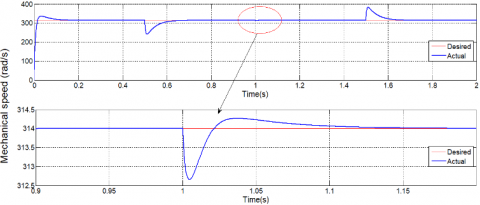Figure 11. Mechanical speed with application of a resistive torque of 6 (N.m) between instants t=0.5s and t=1.5s and Rs increase by 50% with zoom for vector control

## 7.12.pngFigure 12. Mechanical speed with application of a resistive torque of 6 (N.m) between instants t=0.5s and t=1.5s and Rs increase by 50% with zoom for by fuzzy logic control

## 7.13.png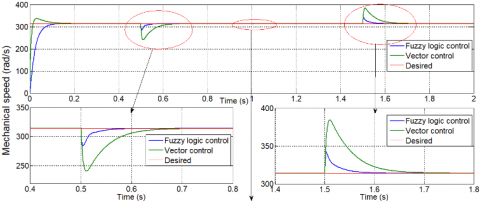## 7.13b.png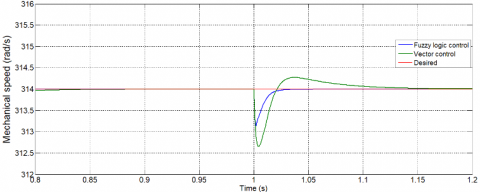Figure 13. Mechanical speed with application of a resistive torque of 6 (N.m) between instants t=0.5s and t=1.5s and Rs increase by 50% for two controls

In this test, we visualized the shape of the velocity for simulation duration TS=2s. The two commands proposed have a strong robustness and ensure good performance even in the presence of small parametric variations with external disturbances; however, fuzzy logic control is the best command with near-smooth velocity at the point of parametric variations of the machine, followed by the vector control which gives us weak ripple.

5. Conclusion

In this paper, we presented a comparative study between two commands applied on the Permanent Magnet Synchronous Machine to represent the efficiency and performance of each during the presence and absence of parametric variations and external variations. This study is based on three essential criteria: qualitative, quantitative and robustness during transient and permanent operation. For this, a modeling of the different components of this system was conducted. These models were used to develop the two control techniques to ensure precise and continuous control of rotor mechanical power generated while ensuring stability, speed of tracking with zero static error. This has resulted in high system efficiency and optimal production quality. The results obtained show that fuzzy logic control is the most efficient and effective control over our system compared to vector control in the presence and absence of parametric and external variations.

References

 Djerioui, A., Houari, A., Ait-Ahmed, M., Benkhoris, M. F., Chouder, A., Machmoum, M. (2018). Grey Wolf based control for speed ripple reduction at low speed operation of PMSM drives. ISA transactions, 74: 111-119. https://doi.org/10.1016/j.isatra.2018.01.012

 Qiao, W., Tang, X., Zheng, S., Xie, Y., Song, B. (2016). Adaptive two-degree-of-freedom PI for speed control of permanent magnet synchronous motor based on fractional order GPC. ISA Transactions, 34: 303-313. https://doi.org/10.1016/j.isatra.2016.06.008

 Li, L.B., Sun, L.L., Zhang, S.Z., Yang, Q.Q. (2015). Speed tracking and synchronization of multiple motors using ring coupling control and adaptive sliding mode control. ISA Transactions, 58: 635-649. https://doi.org/10.1016/j.isatra.2015.07.010

 Chen, S., Luo, Y., Pi, Y. (2015). PMSM sensorless control with separate control strategies and smooth switch from low speed to high speed. ISA Transactions, 58: 650-658. https://doi.org/10.1016/j.isatra.2015.07.013

 Houari, A., Bouabdallah, A., Djerioui, A., Machmoum, M., Auger, F., Darkawi, A., Olivier, J., Benkhoris, M.F. (2018). An effective compensation technique for speed smoothness at low speed operation of PMSM drives. IEEE Trans on Industry Applications, 54(1): 647-655. https://doi.org/10.1109/TIA.2017.2740388

 Wu, L.H., Liu, Z.H., Li, X.H. (2015). GPU-accelerated parallel coevolutionary algorithm for parameters identification and temperature monitoring in permanent magnet synchronous machines. IEEE Transactions on Industrial Informatics, 11(5): 1220-1230. https://doi.org/10.1109/TII.2015.2424073

 Xu, D., Zhang, S., Liu, J. (2013). Very-low speed control of PMSM based on EKF estimation with closed loop optimized parameters. ISA Transactions, 52(6): 835-843. https://doi.org/10.1016/j.isatra.2013.06.008

 Reigosa, D., Fernandez, D., Gonzalez, C., Lee, S.B., Briz, F. (2017). PMSM drive control using analog hall-effect sensors. 2017 IEEE Energy Conversion Congress and Exposition (ECCE), Cincinnati, OH, USA, pp. 1-5. https://doi.org/10.1109/ECCE.2017.8096694

 Song, Z., Sun, K.B. (2014). Adaptive backstepping sliding mode control with fuzzy monitoring strategy for a kind of mechanical system. ISA Transactions, 53(1): 125-133. https://doi.org/10.1016/j.isatra.2013.07.017

 Trabelsi, R., Khedher, A., Mimouni, M.F., M’sahli, F. (2012). Backstepping control for an induction motor using an adaptive sliding rotor-flux observer. Electric Power Systems Research, 93: 1-15. https://doi.org/10.1016/j.epsr.2012.06.004

 Adhikary, N., Mahanta, C. (2013). Integral backstepping sliding mode control for underactuated systems: Swing-up and stabilization of the Cart–Pendulum System. ISA Transactions, 52(6): 870-880. https://doi.org/10.1016/j.isatra.2013.07.012

 Hagras, A. (2018). Nonlinear adaptive extended state space predictive control of PMSM. International Transactions on Electrical Energy Systems, 29(1): 1-15. https://doi.org/10.1002/etep.2677

 Meng, Y.B., Liu, B.Y., Wang, L.C. (2019). Speed control of PMSM Based on an optimized ADRC controller. Mathematical Problems in Engineering, 2019: 1-18. https://doi.org/10.1155/2019/1074702

 Wang, S., Shi, S.S., Chen, C., Yang, G., Qu, Z.J. (2009). Identification of PMSM Based on EKF and Elman Neural Network. Proceedings of the IEEE International Conference on Automation and Logistics, Shenyang, China, pp. 1459-1463. https://doi.org/10.1109/ICAL.2009.5262728

 Rouabhi, R., Djerioui, A. (2014). Control of the power generated by variable speed wind turbine driving a DFIG. Journal of Electrical Engineering, 14(3): 1-7.

 Rouabhi, R., Abdessemed, R., Chouder, A., Djerioui, A. (2015). Power quality enhancement of grid connected doubly-fed induction generator using sliding mode control. International Review of Electrical Engineering, 10(2): 266-276.

 Rouabhi, R., Abdessemed, R., Chouder, A., Djerioui, A. (2015). Hybrid backstepping control of a doubly fed wind energy induction generator. The Mediterranean Journal of Measurement and Control, 11(1): 367-376.

 Celik, H., Yigit, T (2018). Field-oriented control of the PMSM with 2-DOF PI controller tuned by using PSO. International Conference on Artificial Intelligence and Data Processing, Malatya, Turkey. https://doi.org/10.1109/IDAP.2018.8620902

 Sharma, R.K., Sanadhya, V., Behera, L., Bhattacharya, S. (2008). Vector control of a permanent magnet synchronous motor. Annual IEEE India Conference, Kanpur, India, pp. 81-86. https://doi.org/10.1109/INDCON.2008.4768805

 Shi, L.C., Zhang, C., Yue, S.G. (2014). Vector control IC for PMSM. IEEE International Conference on Electron Devices and Solid-State Circuits, Chengdu, China, 1459-1463. https://doi.org/10.1109/EDSSC.2014.7061126

 Sabrine, J., Khaled, N. (2018). A comparative study on the PMSM control system using the nonlinear dynamic inversion method. International Conference on Advanced Systems and Electric Technologies (IC_ASET), Hammamet, Tunisia, pp. 490-494. https://doi.org/10.1109/ASET.2018.8379904

 Chai, S., Wang, L.P., IEEE, Rogers, E., Member, IEEE. (2013). A cascade MPC control structure for a PMSM with speed ripple minimization. IEEE Transactions on Industrial Electronics, 60(8): 2978-2987. https://doi.org/10.1109/TIE.2012.2201432

 Daya, J.L.F., Subbiah, V. (2009). A novel fuzzy logic based robust speed controller for permanent magnet synchronous motor servo drive. TENCON 2009 - 2009 IEEE Region 10 Conference, Singapore, pp. 1-4. https://doi.org/10.1109/TENCON.2009.5395845

 Maamoun, A., Alsayed, Y.M., Shaltout, A. (2013). Fuzzy logic based speed controller for PMSM drive”. IEEE International Conference on Mechatronics and Automation, Takamatsu, Japan, pp. 1518-1522. https://doi.org/10.1109/ICMA.2013.6618139

 Daya, J.F.L., Subbiah, V. (2010). Robust control of sensorless PMSM drive using fuzzy logic. In 2010 2nd International Conference on Advanced Computer Control, Shenyang, China, pp. 14-18. https://doi.org/10.1109/ICACC.2010.5486774

 Seghir, A.N., Henni, T., Azira, M. (2013). Fuzzy and adaptive fuzzy PI controller based Vector control for PMSM. 10th IEEE Inter Conference on Networking, Sensing and Control, Evry, France, pp. 491-496. https://doi.org/10.1109/ICNSC.2013.6548788

 Manukonda, D., Gorantla, S.R. (2018). A fuzzy logic controller based vortex wind turbine for commercial applications. Advances in Modelling and Analysis A, 91(2): 54-58.

 Joy, J., Ushakumari, S. (2018). Performance comparison of a bridge-less canonical switching cell and H-bridge inverter with SVPWM fed PMBLDC motor drive under fuzzy logic controller. Advances in Modelling and Analysis A, 91(4): 193-201.

 Mini, V.P., Ushakumari, S. (2014). Rotor fault detection and diagnosis of induction motor using fuzzy logic. Advances in Modelling and Analysis A, 87(2): 19-40.